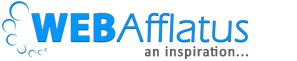# How to plot two graphs in the same plot in R and R ShinyWe as data analyst always have to do basic EDA or data manipulating before sorting the variable for developing any model. One of the first steps that we follow in EDA is bi-variate analysis. I will not go into details how or why we do bi-variate analysis, as you must know already and just want to learn how to plot 2 graphs in the same plot area in R. So let’s get started. I simply wrote an R code with comments to understand each step. Please check out the code below and comment if any step is not clear. Good luck.

```################################################################
################### R Tutorial ###############################

# Purpose: Draw 2 graphs in the same plot using par function
# Author: Deepesh Singh

################################################################

#Creating random dataset
set.seed(199123)

# Creating random population by year
men <- seq (10, 22, by = ((22-10)/(35 - 1)))
income <- sample(25, size = 35, replace = T)
year <- seq(1982,2016)

# Checking the length of each variable to create a dataframe
length(men)
length(income)
length(year)

population_income <- data.frame(year,men,income)

# Now let's plot both variables (men & income) in the same graph

# Setting margin
par(mar= c(5,4,4,5) + 0.1)

# Plot graph with one variable
attach(population_income)
plot(x = year, y = men, col = "blue", type = "l", ylab = "Men population",
main = "Men vs Income over the year")
par(new = T)
# Plot with another variable
plot(x = year, y = income, col = "green", type = "l", xaxt = "n",
yaxt = "n", xlab = "", ylab = "")
axis(4)
mtext("Income by year", side = 4, line = 3)
detach(population_income)

```##### Signature

Deepesh Singh## 3 thoughts on “How to plot two graphs in the same plot in R and R Shiny”

1.Gerardo

Tried to use it in shiny app, but its not working, are there any additional steps to make it work? thanks a lot

2.Anonymous

Works perfectly in R. Exactly what I needed – thank you 🙂

•Deepesh Singh

Welcome 🙂